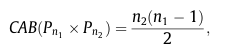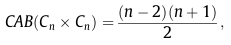# A Hybrid Metaheuristic for the Cyclic Antibandwidth Problem

Lozano, Duarte, Gortázar, Martí (2013)
Optsicom project, University of Valencia (Spain)

# Problem Description

Let G = (V, E) be an undirected graph, where V denotes the set of vertices and E the set of edges. Let n = |V| and m = |E|. A labeling f of the vertices of G is a bijective mapping from V to the set of integers {1, 2, . . . , n}, where each vertex v in V receives a unique label f(v) in {1, 2, . . . , n}. A circular arrangement of a labeling, simply called circular labeling, arranges the vertices of the graph in a cycle Cn where the last vertex (the one with label n) is next to the ﬁrst vertex (the one with label 1).

Given a circular labeling f, let us deﬁne the clockwise distance d+(u, v) = |f(u) − f(v)| with (u, v) in E and, similarly the counterclockwise distance d(u, v) = n − |f(u) − f(v)| with (u, v) in E. Then, for a given circular labeling f, the cyclic antibandwidth of G, referred to as CAB(G, f), is computed as follows:

CAB(G, f) = min{d+(u,v), d-(u,v) : (u,v) in E}

# State of the Art Methods

Exact results for the CAB problem are proved for some specific classes of graphs like paths, cycles, two dimensional meshes (Cartesian product of two paths), tori (Cartesian product of two cycles), and asymptotic results are obtained for hypercube graphs. Dobrev et al. extended these results to the case of Hamming graphs (Cartesian product of d-complete graphs). However, to the best of our knowledge, only one metaheuristic approach has been presented for finding the cyclic antibandwidth of general graphs, the memetic algorithm by Bansal and Srivastava (2011).

# Instances

In this section several instance sets are described. These intance set have been used in the related literature to compare the performance of methods for the Cyclic Antibandwidth Problem:

• Instances with known optimum
• Paths (Download). This data set consists of 24 graphs constructed as a linear arrangement of vertices such that every vertex has a degree of two, except the first and the last vertex that have a degree of one. The size of these instances ranges from 50 to 1000. For a path Pn with n vertices, S ́kora et al. (2005) proved that the optimal CAB value is:• Cycles (Download). This data set consists of 24 graphs constructed as a circular arrangement of vertices such that every vertex has a degree of two. The size of these instances ranges from 50 to 1000. For a cycle Cn with n vertices the optimal cyclic antibandwidth is• Grids (Download). This data set consists of 24 graphs constructed as the Cartesian product of two paths Pn1 and Pn2. The size of these instances ranges from 81 to 1170. They are also called two dimensional meshes. For a grid Pn1 × Pn2 with n = n1 · n2 vertices the optimal cyclic antibandwidth fulfills that:if n1 is even and n2 is odd (n1 ≥ n2 ), andotherwise.
• Toroidal grids (Download). This data set consists of 37 graphs constructed as the Cartesian product of two cycles (i.e., Cn × Cn ). The size of these instances ranges from 16 to 1600. The optimal cyclic antibandwidth isif n is even, andif n is odd.
• Hamming graphs (Download). This data set consists of 24 graphs constructed as the Cartesian product of d complete graphs Knk , for k = 1, 2, ..., d . The size of these instances ranges from 80 to 1152. The vertices in these graphs are d-tuples (i1, i2, ..., id), where ik in {0, 1, ..., nk−1 }. Two vertices (i1, i2, ..., id) and (j1, j2, ..., jd) are adjacent if and only if the two tuples differ in exactly one coordinate. These graphs are referred to as Hamming graphs. Dobrev et al.  proved that if d ≥ 2 and 2 ≤ n1n2 ≤ ... ≤ nd , then the optimal cyclic antibandwidth for this type of instances is given by:andwhere nd−2 = nd−1 = n, d ≥ 3 and q is the minimal index such that qd − 2 and nq = nd.
• Instances with conjecture optimum
• Three-dimensional meshes (Download). They are 20 graphs defined by the Cartesian product of three paths Pn1 x Pn2 x Pn3 with n = n1 x n2 x n3. The size of these instances ranges from 12 to 3600.
• Double stars (Download). A double star s(n1,n2) consists of two stars K1;n1 and K1;n2 with one edge in common. We generated 20 instances whose size ranges from 20 to 180.
• Hypercubes (Download). The hypercube Qd of dimension d is a d-regular graph with 2d vertices and d·2d-1 edges. Each vertex is labeled by a distinct d-bit binary string, and two vertices are adjacent if they differ in exactly one bit. 7 instances where obtained, ranging from 16 to 1024 vertices.
• Instances with unknown optimum
• Random connected graphs (Download). These graphs are generated by including each of |V|2 possible edges independently with probability pd. Through the experiments, we fixed the parameter pd such that different edge densities are taken into account. The procedure for building these graphs ensures that they are connected.
• Caterpillars (Download). This data set consists of 40 graphs. Each caterpillar, Pn1,n2 is constructed using the path Pn1 and n1 copies of the path Pn2 (usually referred to as “hairs”), where each vertex i in Pn1 is connected to the first vertex of the i-th copy of the path Pn2. The size of these instances ranges from 20 to 1000.
• Complete binary trees (Download). This data set consists of 24 trees, where every tree-level is completely filled except possibly the last level and all nodes are as far left as possible. The size of these instances ranges from 30 to 950.
• Harwell-Boeing Graphs (Download). This data set consists of 24 instances (12 smaller and 12 larger) derived from the Harwell-Boeing Sparse Matrix Collection. Graphs are derived from these matrices as follows. Let Mij denote the element of the i-th row and j-th column of the n×n sparse matrix M. The corresponding graph has n vertices. Edge (i, j) exists in the graph if and only if Mij is not equal to 0. These instances were used in Duarte et al. (2010) with name Harwell-Boeing.

# Computational Experience

We have performed an intensive experimentation with the best known heuristic to compute the best known values for the instances. We have also reviewed related literature searching for better values than these. The final best known values for the instances can be found in this Excel file. All experiments were performed within a time limit of 150 seconds.

# References

• Dobrev, S., R. Kralovic, D. Pardubska, L. Torok, and I. Vrto. Antibandwidth and cyclic antibandwidth of Hamming graphs. Electronic Notes in Discrete Mathematics, 34:295–300, 2009.
• Duarte, A. and R. Martí, M.G.C. Resende and R.M.A. Silva. GRASP with Path Relinking for the Antibandwidth Problem", Networks. In press, 2010.
• R. Bansal, K. Srivastava, A memetic algorithm for the cyclic antibandwidth maximization problem, Soft Computing 15 (2) (2011) 397–412.
• O. Sýkora, L. Torok, I. Vrt’o, The cyclic antibandwidth problem, Electronic Notes in Discrete Mathematics 22 (2005) 223–227.
• Raspaud, A.,, H. Schroder, O. Sykora, L. Torok, and I. Vrto. Antibandwidth and cyclic antibandwidth of meshes and hypercubes. Discrete Mathematics, 309: 3541–3552, 2009.